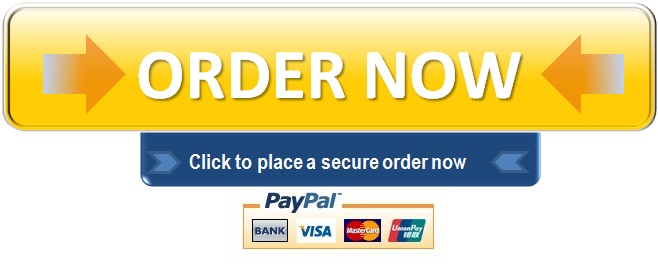# Assessment 2, Question 2

Question 2

A).

Below is a step by step process for finding the incremental cash flows for the project

The initial investment

 \$                                                   \$ Construction of new building                                                                                           25,000,000 Machinery : Purchase cost                                                     14,000,000 Shipping cost                                                          500,000 Installation charge                                                  500,000                                 15,000,000 Initial investment                                                                                                             40,000,000

NOTE: The \$150,000 cost of market research has been left out in the calculations because it is a sunk cost. These are costs already spent and whether the project is implemented or not implemented does not affect that fact (Gitman and Zutter, 2012).Similarly, the \$ 2,000,000 cost of the land has been left out because it is not an opportunity cost. The company already owns the land in addition to the lack of evidence of any best alternative use of the land were the proposal for the new project to be rejected.

Yearly operating cash inflows

 \$ Sales revenue(60,000*510)                                                                                              30,600,000 Expenses(Excluding depreciation): Variable costs(60,000*100)                                                6,000,000 Fixed costs                                                                        15,000,000               (21,000,000) Earnings before depreciation, interest and taxes(EBDIT)                                                 9,600,000 Depreciation(                                                                                    (3,900,000) Earnings before interest and taxes(EBIT)                                                                          5,700,000 Taxes (EBIT*30%)                                                                                                         (1,710,000) Net operating profit after taxes (NOPAT)                                                                         3,990,000 Depreciation                                                                                                                       3,900,000 Operating cash inflows                                                                                                      7,890,000

Terminal cash flows

Since the plant would be fully depreciated at the end of the year, the terminal cash flow will simply be the salvage value which is just \$ 1,000,000.

Summary of cash flows

 End of year Cash Flow 0 (\$40,000,000) 1 \$7,890,000 2 \$7,890,000 3 \$7,890,000 4 \$7,890,000 5 \$7,890,000 6 \$7,890,000 7 \$7,890,000 8 \$7,890,000 9 \$7,890,000 10 \$8,890,000**

**= (\$7,890,000+\$1,000,000)

B).
i). Net Present Value
The NPV is this case is the sum of three present values viz the initial cash outflow of \$40,000,000, the \$7,890,000 annuity from year 1-9 and the \$8,890,000 at the end of year 10.The initial cash outflow does not need any discounting.

The PV of the \$7,890,000 annuity is given by the formula below
〖PV〗_n=(CF/r)×[1-1/(1+r)^n ]
Where:
〖PV〗_n=Present value of an annuity=?
CF=Cash flow=\$7,890,000
r=required rate of return=15%
n=number of periods=9

〖PV〗_n=(\$7,890,000/0.15)×[1-1/(1+0.15)^9 ]
=(\$7,890,000/0.15)×[1-1/3.517876292]
=\$37,647,797.13

The PV of \$8,890,000 at the end of year 10 is given by
PV=〖FV〗_n/〖(1+r)〗^n

Where:
PV=Present value
〖FV〗_n=\$8,890,000
r=15%
n=10

PV=\$8,890,000/〖(1+0.15)〗^10
=\$2,197,472.04

The NPV is, therefore, the sum of the three as given below:
NPV={\$(37,647,797.13+2,197,472.04)-\$40,000,000}
=(\$154,730.83)

ii).
The Internal Rate of Return (IRR)
Under the interpolation method, we need two NPVs at different discount rates. One should be negative while the other should be positive. As we already have an NPV at a 15% discount rate, we need to calculate another NPV at say 10% discount rate as shown below.
The NPV is this case is the sum of three present values viz the initial cash outflow of \$40,000,000, the \$7,890,000 annuity from year 1-9 and the \$8,890,000 at the end of year 10.The initial cash outflow does not need any discounting.

The PV of the \$7,890,000 annuity is given by the below formula
〖PV〗_n=(CF/r)×[1-1/(1+r)^n ]

Where:
〖PV〗_n=Present value of an annuity=?
CF=Cash flow=\$7,890,000
r=required rate of return=10%
n=number of periods=9

〖PV〗_n=(\$7,890,000/0.10)×[1-1/(1+0.10)^9 ]
=(\$7,890,000/0.10)×[1-1/2.357947691]
=\$45,438,697.91

The PV of \$8,890,000 at the end of year 10 is given by
PV=〖FV〗_n/〖(1+r)〗^n

Where:
PV=Present value
〖FV〗_n=\$8,890,000
r=10%
n=10

PV=\$8,890,000/〖(1+0.10)〗^10
=\$3,427,479.843
The NPV is, therefore, the sum of the three as given below:
NPV={\$(45,438,697.91+3,427,479.843)-\$40,000,000}
=\$8,866,177.753

With both NPVs, we can then proceed to estimate the IRR using the formula below:

IRR=R_1+((R_2-R_1)×〖NPV〗_1)/((〖NPV〗_1-〖NPV〗_2))
Where:
R_1=Lower discount rate=10%
R_2=Higher discount rate=15%

〖NPV〗_1=NPV calculaterd using lower discount rate=\$8,866,177.753
〖NPV〗_2=NPV calculated using higher discount rate=(\$154,730.83)
IRR=0.10+((0.15-0.10)×\$8,866,177.753)/((\$8,866,177.753+\$154,730.83))

=0.10+443,308.8877/9,020,908.583

IRR≅14.9142%

iii).

Payback Period (PP)

This is the period that it takes to recover the initial investment in a project. Below is a detailed calculation of the PP for the project.

 Year Cash Flow Cumulative Cash Flow 0 (\$40,000,000) (\$40,000,000) 1 \$7,890,000 (\$32,110,000) 2 \$7,890,000 (\$24,220,000) 3 \$7,890,000 (\$16,330,000) 4 \$7,890,000 (\$8,440,000) 5 \$7,890,000 (\$550,000) 6 \$7,890,000 \$7,340,000 7 \$7,890,000 \$15,230,000 8 \$7,890,000 \$23,120,000 9 \$7,890,000 \$31,010,000 10 \$8,890,000 \$39,900,000

iv).

Discounted Payback Period

 Year(n) Cash Flow Discounted Cash Flow(CF/(1+0.15)n Cumulative  Discounted Cash Flow 0 (\$40,000,000) (\$40,000,000) (\$40,000,000) 1 \$7,890,000 \$6,860,869.565 (\$33,139,130.44) 2 \$7,890,000 \$5,965,973.535 (\$27,173,156.9) 3 \$7,890,000 \$5,187,803.074 (\$21,985,353.83) 4 \$7,890,000 \$4,511,133.108 (\$17,474,220.72) 5 \$7,890,000 \$3,922,724.442 (\$13,551,496.28) 6 \$7,890,000 \$3,411,064.732 (\$10,140,431.54) 7 \$7,890,000 \$2,966,143.245 (\$7,174,288.299) 8 \$7,890,000 \$2,579,254.996 (\$4,595,033.303) 9 \$7,890,000 \$2,242,830.431 (\$2,352,202.872) 10 \$8,890,000 \$2,197,472,037 (\$154,730.835)

With a cumulative discounted cash flow of (\$154,730.835) at the end of 10 years, this project does not have a discounted payback Period.

To begin with, the \$2,000,000 involved in purchasing the land 3 years ago should not be included in the calculation of the initial investment. The company already owns this land. There is no indication that this land was meant for any other project. As a result, there are no alternatives that are going to be put at halt for the sake of the new project. This means that the \$2,000,000 used in the purchase of the land 3 years ago cannot be considered as an opportunity cost hence should not be included in the calculation of the initial investment.

Based on the above calculations, the project should not be undertaken. The project’s NPV is (\$154,730.83). As a decision rule, any project with a NPV<0 should be rejected. The IRR of the project is also 14.9142%. The required rate of return for the project is 15%. Investment in a project is only feasible if the internal rate of return (IRR) is greater than the required rate of return. In this case, IRR is less than the required rate of return, and therefore the project should not be undertaken.

The payback period is not favorable in making the decision in this case because it ignores time value of money and the benefits that would accrue after the payback period.

Reference

Gitman, L.J. and Zutter, C.J. (2012). Principles of Managerial Finance.13th ed.Boston, MA:         Pearson.

Do you need an Original High Quality Academic Custom Essay?